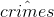### Create an Account

Home / Questions / Suppose that you are interested in estimating the link between the unemployment rate (unem...

# Suppose that you are interested in estimating the link between the unemployment rate (unem), size of the city population (pop), and number of crimes (crimes). The following equation has been estimated

Suppose that you are interested in estimating the link between the unemployment rate (unem), size of the city population (pop), and number of crimes (crimes). The following equation has been estimated using data on U.S. cities:

log() = −2.13 + 0.001unem + 0.98 log(pop), n = 92, R2 = 0.854.

a. Interpret the coefficient on unem. Be specific.

b. Interpret the coefficient on log(pop). Be specific.

c. What are the units of measurement for the R2 in this regression (number of crimes? percentage points? or is R2 unit-free)? Explain.

May 06 2021 View more View LessSubscribe To Get Solution